Courses

# Chapter 2 Thyristor - Notes, Power Electronics, Electrical Engineering Electrical Engineering (EE) Notes | EduRev

## Electrical Engineering (EE) : Chapter 2 Thyristor - Notes, Power Electronics, Electrical Engineering Electrical Engineering (EE) Notes | EduRev

The document Chapter 2 Thyristor - Notes, Power Electronics, Electrical Engineering Electrical Engineering (EE) Notes | EduRev is a part of the Electrical Engineering (EE) Course Electrical Engineering SSC JE (Technical).
All you need of Electrical Engineering (EE) at this link: Electrical Engineering (EE)

THYRISTOR

THYRISTOR = Thyratron + Transistor

• Thyristor is a family of devices, construction wise similar to the transistor but function as Thyratron tube 
• The most important and most widely used member of thyristor family is the SCR (silicon controlled rectifier)

SCR

It is four layer, three junction device, having three terminals namely anode (A), cathode (K)  and gate (G) as shown in figure below: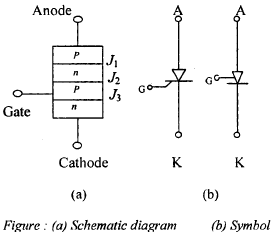Static characteristic of thyristors

The characteristic of SCR can be classified into three modes.
1. Reverse blocking mode
2. Forward blocking mode
3. Forward conducting mode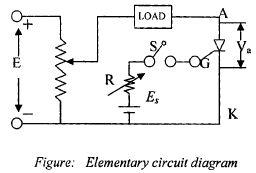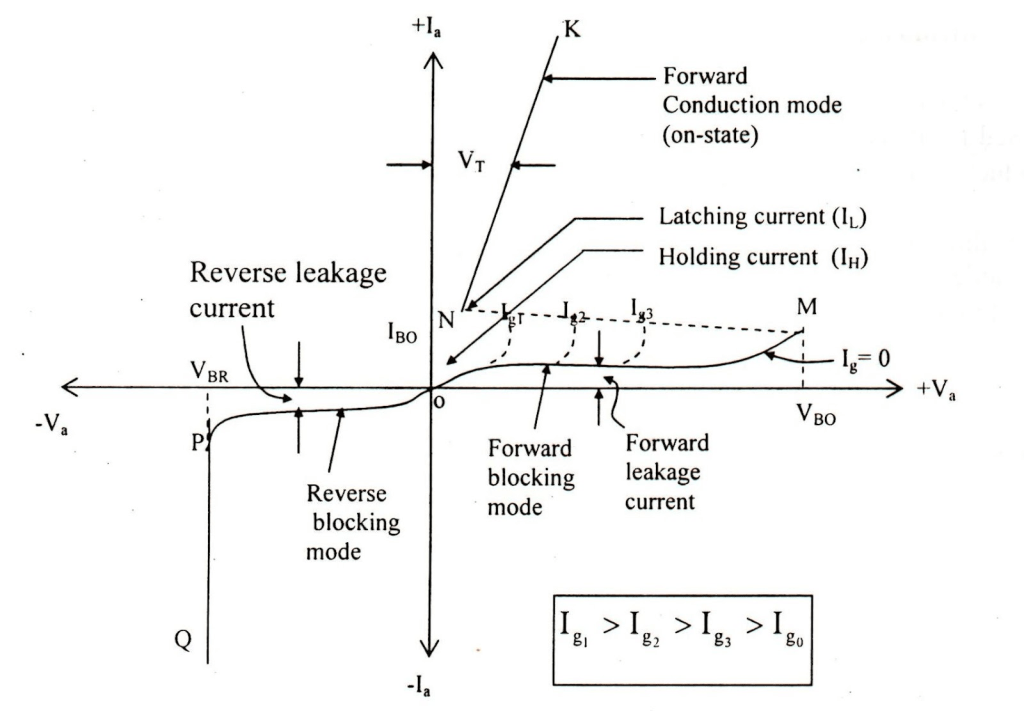where,

VBO = Forward break over voltage
VBR = Reverse break over voltage
Ig = Gate current

(1) Reverse Blocking Mode

In elementary circuit diagram, if A is made negative w.r.t. K i.e. Va = – ive junction J1 and J3 are reverse biased while J2 is forward bias.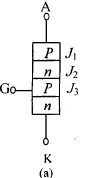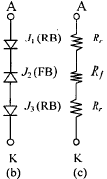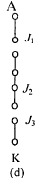• SCR appear like three diodes are connected as shown in figure (b). As J1 and J2 are R.B, so offer high resistance Rr (ideally open circulated), while that of J2 being F.B. offers very low resistance Rf (ideally short circuited.)
• A very small leakage current flow (like reverse leakage current of diode) in the circuit.
• As reverser voltage Va (– ive) is increased further at a particular value of voltage say VBR (– ive)reverse biased junction Jand J3 breakdown hence current rises sharply, (the ch. represented in the IIIrd quadrant with load resistance  to zero in the characteristics.
• VBR is known as reverse break over voltage.

(2) Forward Blocking Mode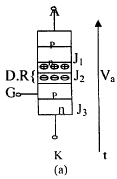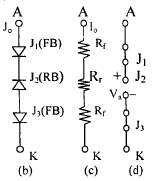• If Anode (A) is made positive w.r.t. Cathode (K) i.e., Va = + ive, junction Jand J3 are, forward biased while J2 is reverse biased. So J2 offers very high resistance Rr (ideally open circuited seee figure (c) and (d) so junction J2 block the current even when SCR is forward biased but very small leakage current IO (i.e. forward leakage current flows) flow in the CKT. So SCR has the capability to block the current vern in forward biased condition called “Forward Blocking Mode”
• As stated above if Va ≥ VBO, J2 breakdown but this method generally not employed. Forward break down voltage can be decreased if positive gate current is made to flow.
• In conduction mode the Anode current Ia is mainly decided by the load present in the circuit. The very small voltage drop exist there, across the SCR i.e. known as On-state voltage drop varies between 1-1.5 V.
• As ideally J2 is open whole of  Va appears across J2 so if  Va is increased to or beyond a particular value VBO (i.e,. forward break over voltage) J2 breakdown and start conduct the forward current, so SCR jumps into forward conduction mode.

Two Transistor Analogy of A Thyristor

The working of thyristors may be analysed as back-to-back connected two transistors (one NPN and one PNP). Figure below shows such as representation. Q1 is PNP and Q2 is NPN transistor. Collector of Q1 is connected to base of Q2 and vice-versa.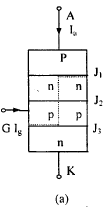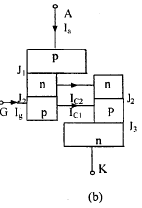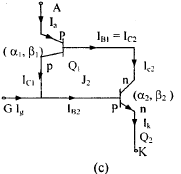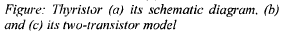By KCL, IA = IC1 + IC2 ......(i)

IC1 = β1IC2 + β1ICO1 + ICO1 ......(ii)

IC2 = β2IC1 + β2ICO2 + ICO2......(iii)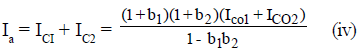For zero gate current applied  β1,  β2 are very small and hence

Ia = ICO1 + ICO2    .............(v)

When once Ia has built up in circuit, opening of the switch in the gate circuit has no influence. The only way to turn off the thyristor is to disconnect it from the supply or to decrease the anode current below a certain value.

Thyristor Turn-on-Methods:

When SCR is forward biased (FB) it can be turned on by

1. Forward Voltage Triggering 

• As stated earlier almost whole of the Va appears across the reverse biased junction J2. 
• If Va is increased the reverse voltage across J2 increases and at Va = VBO avalanche break down occur and  J2 breakdown. Hence SCR starts conducting 
• This method generally not employed in practice as it required a higher voltage for triggering than the normal working voltage as well as it may damage the SCR.

2. [dv/dt] Triggering:

• In F.B. SCR the region around J2 (space charge region or depletion region) acts as an insulator while on both sides of this region, charge carriers are trapped so act as conductor. This arrangement appear like a capacitor.
• Charging current in capacitor i = [d/dt] (CjVa) = Cj [dVa/dt] Where, CJ = Junction capacitance 
• If [dVa/ dt] rate is more than the permitted value (generally 20-500 V/μs), significant current flows which may turn on thw SCR
• To avoid this false triggering due to high [dVa/ dt] (Which occurs when supply is switched on )snubber circuit is used

3. Temperature triggering During forward  blocking, most of the applied voltage appears across reverse junction J2. This voltage across junction J2 associated with leakage current may raise the temperature, leakage current through junction J2 furthers increases. This cumulative process may turn on the SCR at some high temperature.

(4) Light Triggering 

• The surface of inner p-layer is made photosensitive.
• When pulse of light of appropriate wave length and of sufficient intensity is irradiated, charges carrier (holes and electrons) are  generated in inner p-layer thus width of depletion region in inner p-layer gets reduced, hence avalanche break occur may turn ON SCR.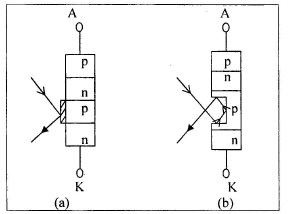• To utilize the light more, a recess (or niche) is provide in the inner p-plane. 
• Light Activated SCR (LASCR) is used in conjunction with gate triggering. 
• LASCR provide electrical isolation between power circuit (Anode-circuit) and control circuit (Firing circuit)  When Gate G is made + ive circuit w.r.t. cathode (k), the gate current established from G and k. Thus, inner p-layer is flooded with electons, supplied from outer n-layer (as it is heavily doped).
• Turuing on of SCR by gate triggering is simple reliable and efficient, it is there for most usual method of the forward biased SCR.

(5) Gate Triggering

• As SCR being F.B some of these electorns reach to the depletion region, thus reducing the width of D.R. in p-layer see figure. so breakdown occurs at lower voltage than VBO.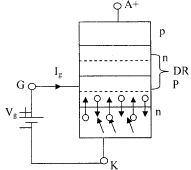• Once SCR is in ON-state no IR is required, but IR should not be reduced to zero before the anode current attains a certain value called Latching current. So lathing current is defined as the minimum value of anode current which it must attain to remain in conduction even after removal of gate signal.
• Once SCR is ON. To turn it off, Anode current must be reduced below certain current called defined as the value of anode current below which it must fall to turn-off SCR.

Ilatching > Iholding
Assumed that
Ilatching = 3* Iholding

SCR Protection

[di/dt] protection

• When a thyristor is forward biased and is turned on by a gate pulse, conduction of anode current begins in the immediate neighborhood of the gatecathode junction. Thereafter, the current spreads across the whole area of junction. The thyristor design permits the spread of conduction to the whole junction area as rapidly as possible. However, if the rate of rise of anode current, i.e. [di/ dt], is large as compared to the spread velocity of carriers, local hot spots will be formed near the gate conduction on account of high current density. This localized heating may destroy the thyristor. 
• To limit [di/dt] with in safe limit (typical value 20-500 A/μs) and inductor is connected in series. 
• Local spot heating can also be avoided by ensuring that the conducting spreads to the whole area as rapidly as possible.

2.[dv/dt] protection 

• In FB SCR the region around J2 (space charge region or depletion region) acts as an insulator while on both sides of this region, charge carriers are trapped so act as conductor this arrangement appear like a capacitor known as junction capacitance Cj
• Charging current in capacitor.
i = [d/dt] (CjVa) = Cj [dVa/dt]
• If the rate of forward voltage [dVa/dt] is high, the charging current i will be more. This charging current plays the role of gate current and turns on the SCR even when gate signal is  zero. Such phenomena of turning on a thyristor called dv/dt turn and must be avoided as it leads to false operation of the thyristor circuit.

3. Over voltage protection

• SCRs are often subjected  to the internal and external over voltage, to suppress the effect to over voltage, voltage clamping device resistor (VCD and VCR) or simply non linear resistor is connected across SCR.
• From figure is clear that resistance of this device decreases as voltage increases hence in normal voltage condition they behave as open circuit while becomes shorted in high voltage condition.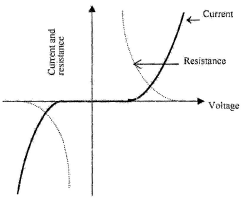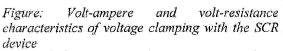4) Over current protection: 

• It is provide using over current relays or fuses.
• Other efficient scheme for this purpose is Electronic Crowbar Protection.
• Crow bar thyristor possesses high surge current capability.
• When current in the circuit ends the safe value. Voltage exceeds across sensing resistor. So gate circuit turn on the crowbar thyristor instantly thus protecting the circuit.

5. Gate protection Stabilized gate voltage is obtained using zener diode, while R1 and C1 are used as a filter.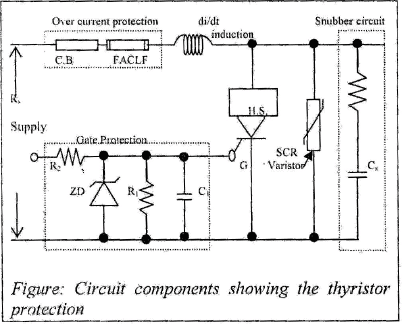Thyristor Triggering Circuits Out of the various method of turning on a thyristor described earlier, gate signal method is widely used.
Some triggering or firing circuits are described as follows:

1. Direct Current Firing A continuous gate signal is not generally requirec except in applications where the thyristor may turn, off before the time required. Figure shows a typicai d.c. firing signal circuit. When the anode is positive and the thyristor is on, the gate current is reduced as the voltage to produce the gate current no^ corresponds to the forward voltage drop of the thyristor, which is about 1 V. The diode D is connected between the gate and the cathode are prevents large reverse voltage across the gate and the cathode terminals when the current is interrupted.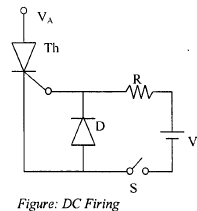2. AC Signal Firing

This is knows as phase control of firing and the instant of firing can be manipulated to occur at any particular-phase between 0 to n of the half cycle of an AC signal.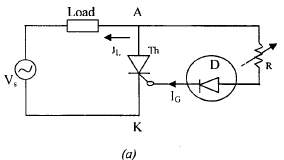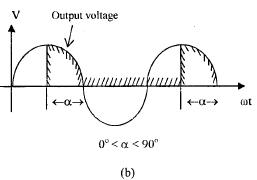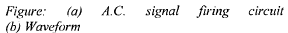Phase control is used to alter AC power to be supplied to load. The power is modulated by varying the part of the voltage cycle during which the current is allowed to conduct. To achieve control, the pulse to turn of the thyristor at the correct part of the cycle is synchronized with the AC supply and its phase with respect to the supply voltage is controlled. The anode to cathode phase control can have any desired angle for conduction e.g. 90º, 120º, 150º etc. Figure (b) shows a simple circuit to obtain 90º phase control.
A variable resistance R and a diode D is connected between anode and the gate across the thyristor The alternating current supply is connected to thyristor and load. By varying the resistance, the delay time is adjusted. Increased resistance delays the time or the phase in the cycle so that the voltage is positive enough to drive sufficient gate current to turn the thyristor on.

3. Pulse Firing There are advantages in having pulse signals rather than continued DC. signals. Pulse signals may be single or multiple pulses which can be controlled in times: otherwise alternatively they may be on-off pulses, figure below shows a simple circuit giving on-off pulse signals.
This circuit uses saturable core transformer. When the anode is positive, the thyristor will turn on if the transformer is unsaturable. When the switch is closed, the transformer will get saturated and the thyristor will not fire.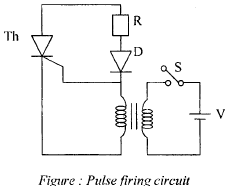4. UJT Firing A uni-junction transistor circuit used for tiring of a thyristor is as shown in figure. Capacitor C1 charges by R1 C1 combination with UJT breaks down and the capacitor C1 discharges through pulse transformer connected with the gate.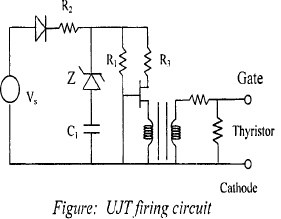Offer running on EduRev: Apply code STAYHOME200 to get INR 200 off on our premium plan EduRev Infinity!

65 docs|37 tests

,

,

,

,

,

,

,

,

,

,

,

,

,

,

,

,

,

,

,

,

,

,

,

,

,

,

,

;